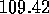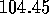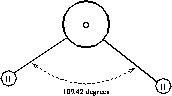Next: Simulations with the Up: Theory Previous: The Coulomb potential

## The SPC water model

To calculate the interactions between molecules in liquid water, we need a model of the individual water molecule that tells us where the charges reside. In the Wasser simulation program we use a very simple model to represent the water molecule called the SPC model. In the SPC, or Simple Point Charge model, the water molecule has three centers of concentrated charge: a predominance of positive charge on the H--atoms and excess negative charge on the O--atom. Our assumption that there are point charges is an approximation that leads to an incorrect value for the permanent dipole moment of the water molecule. To correct this, the H--O--H bond angle is changed todegrees in the model (compared with the experimentally--found H--O--H bond angle ofdegrees.) Fig. 3.7.1.Fig. 3.7.1 The SPC--water molecule. To yield overall neutrality, the negative partial charge on the oxygen atom has twice the magnitude of the partial positive charge on each of the two hydrogen atoms.

The results of the charge concentration and the widened V--shaped bond angle is that the permanent dipole moment of the SPC--model water molecule has a value close to that measured in experiment. It also has an effect on the mobility of the molecules. The molecule is able to move faster than it would in ``real'' water due to the missing two lone electron pairs in the SPC--model. This effect however decreases when the temperature is increased.

In the Wasser program the oxygen atoms are represented by a red color, the hydrogen atoms by a white color. The hydrogen bonds are represented by a turquoise colored ``stick'' between an oxygen and a hydrogen.Next: Simulations with the Up: Theory Previous: The Coulomb potential